# Texas Go Math Grade 8 Lesson 12.1 Answer Key Properties of Translations

Refer to our Texas Go Math Grade 8 Answer Key Pdf to score good marks in the exams. Test yourself by practicing the problems from Texas Go Math Grade 8 Lesson 12.1 Answer Key Properties of Translations.

## Texas Go Math Grade 8 Lesson 12.1 Answer Key Properties of Translations

Explore Activity 1

Exploring Translations

You learned that a function is a rule that assigns exactly one output to each input. A transformation is a function that describes a change in the position, size, or shape of a figure. The input of a transformation is the preimage, and the output of a transformation is the image.A translation is a transformation that slides a figure along a straight line. The image has the same size and shape as the preimage.

The triangle shown on the grid is the preimage (input). The arrow shows the motion of a translation and how point A is translated to point A’.A. Trace triangle ABC onto a piece of paper. Cut out your traced triangle.
B. Slide your triangle along the arrow to model the translation that maps point A to point A’.
C. The image of the translation is the triangle produced by the translation. Sketch the image of the translation.
D. The vertices of the image are labeled using prime notation. For example, the image of A is A’. Label the images of points B and C.
E. Describe the motion modeled by the translation.
Move ________ units right and ________ units down.
F. Check that the motion you described in part E. is the same motion that maps point A onto A’, point B onto B’, and point C onto C.

Reflect

Question 1.
How is the orientation of the triangle affected by the translation?
The image and the preimage of the triangle have the same orientation.
The orientation doesn’t change.

Explore Activity 2

Properties of Translations

Use trapezoid TRAP to investigate the properties of translations.A. Trace the trapezoid onto a piece of paper. Cut out your traced trapezoid.
B. Place your trapezoid on top of the trapezoid in the figure. Then translate your trapezoid 5 units to the left and 3 units up. Sketch the image of the translation by tracing your trapezoid in this new location. Label the vertices of the image T’, R’, A’, and P’.
C. Use a ruler to measure the sides of trapezoid TRAP in centimeters.
TR = ___________ RA = ___________ AP = ___________ TP = ___________
D. Use a ruler to measure the sides of trapezoid T’R’A’P’ in centimeters.
T’R’ = ___________ R’A’ = ___________ A’P’ = ___________ T’P’ = ___________
E. What do you notice about the lengths of corresponding sides of the two figures?
F. Use a protractor to measure the angles of trapezoid TRAP.
m∠T = ___________ m∠R = ___________ m∠A = ___________ m∠P = ___________.
G. Use a protractor to measure the angles of trapezoid T’R’A’P’.
m∠T’ = ___________ m∠R’ = ___________ m∠A’ = ___________ m∠P’ = ___________.
H. What do you notice about the measures of corresponding angles of the two figures?
I. Which sides of trapezoid TRAP are parallel? How do you know?
Which sides of trapezoid T’R’A’P’ are parallel?.
What do you notice?

Reflect

Question 2.
Make a Conjecture Use your results from parts E, H, and I to make a conjecture about translations.
The size, shape and orientation stays the same after translations.

Question 3.
What can you say about translations and congruence?
A translation produces a figure that is congruent to the original figure.

Question 4.
The figure shows parallelogram ABCD. Graph the image of the parallelogram after a translation of 5 units to the left and 2 units down.Step 1: Translate point A.
Count left 5 units and down 2 units and plot point E(-5, 1).

Step 2: Translate point B.
Count left 5 units and down 2 units and plot point F(-2, 1)

Step 3: Translate point C.
Count left 5 units and down 2 units and plot point G(0, -3).

Step 4: Translate point D.
Count left 5 units and down 2 units and plot point H(-3, -3).

Step 5: Connect E, F, G and H to form parallelogram EFGH.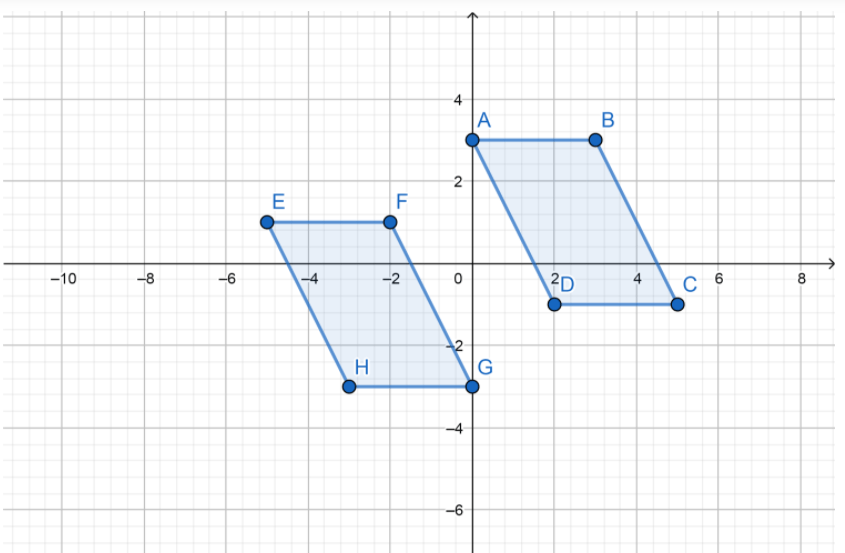E(-5, 1)
F(-2, 1)
G(0, -3)
H(-3, -3)

Question 1.
Vocabulary A __________ is a change in the position, size, or shape of a figure.
A transformation is a change in the position, size, or shape of a figure.

Question 2.
Vocabulary When you perform a transformation of a figure on the coordinate plane, the input of the transformation is called the __________, and the output of the transformation is called the ___________.
When you perform a transformation of a figure on the coordinate plane, the input of the transformation is called the preimage, and the output of the transformation is called the image.

Question 3.
Joni translates a right triangle 2 units down and 4 units to the right. How does the orientation of the image of the triangle compare with the orientation of the preimage? (Explore Activity 1)
A translation doesn’t change the shape and size of a geometric figure.
This means that two triangles are identical in shape and size, so they are congruent and the orientation is the same.

Question 4.
Rashid drew rectangle PQRS on a coordinate plane. He then translated the rectangle 3 units up and 3 units to the left and labeled the image P’Q’R’S’. How do rectangle PQRS and rectangle P’Q’R’S’ compare? (Explore Activity 2)
A translation doesn’t change the shape and size of a geometric figure.
This means that two rectangles are identical in shape and size, so they are congruent.

Question 5.
The figure shows trapezoid WXYZ. Graph the image of the trapezoid after a translation of 4 units up and 2 units to the left. (Example 1)Step 1: Translate point W.
Count up 4 units and left 2 units and plot point E(-4, 3).

Step 2: Translate point X.
Count up 4 units and left 2 units and plot point F(2, 3).

Step 3: Translate point Y.
Count up 4 units and left 2 units and plot point G(1, 1).

Step 4: Translate point Z.
Count up 4 units and left 2 units and plot point H(-3, 1).

Step 5: Connect E, F, G and H to form trapezoid EFGH.E(-4, 3)
F(2, 3)
G(1, 1)
H(-3, 1)

Essential Question Check-In

Question 6.
What are the properties of translations?
A translation is a geometric transformation that moves every point of a figure for a space by the same amount in a given direction.
This means that the figures are identical and congruent.

Question 7.
The figure shows triangle DEF.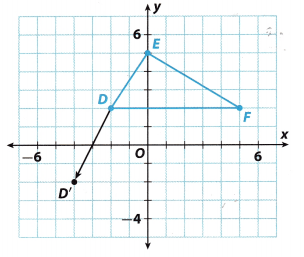a. Graph the image of the triangle after the translation that maps point D to point D’.
From the photo, we can see that point D has been moved 4 units down and 2 units to the left. On the same way, we move the other points.
Step 1: Translate point E.
Count down 4 units and left 2 units and plot point E'(-2, 1).

Step 2: Translate point F.
Count down 4 units and left 2 units and plot point F'(3, -2).

Step 3: Connect D’, E’ and F’ to form triangle D’E’F’.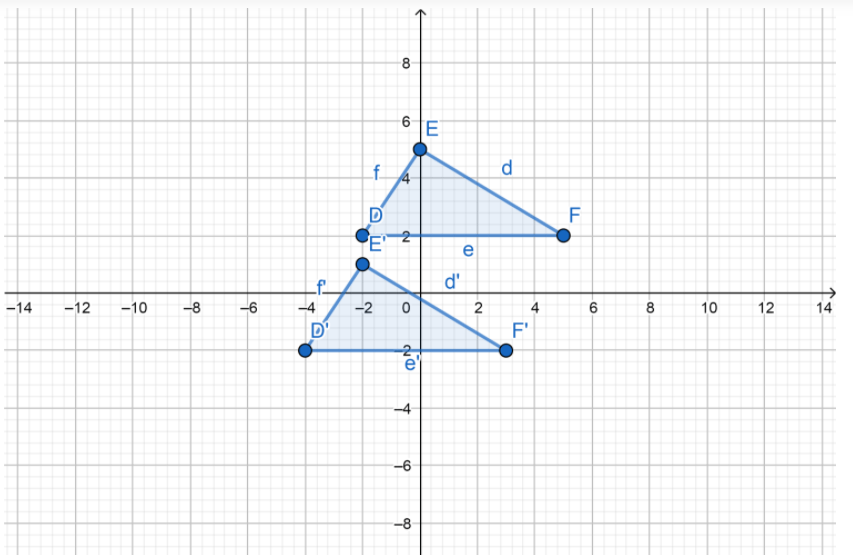b. How would you describe the translation?
With a translation, we moved the triangle 4 units down and 2 units to the left.

c. How does the image of triangle DEF compare with the preimage?
The preimage has the same size, orientation and shape, but a different location from the image.

Question 8.
a. Graph quadrilateral KLMN with vertices K(-3, 2), L(2, 2), M(0, -3), and N(-4, 0) on the coordinate grid.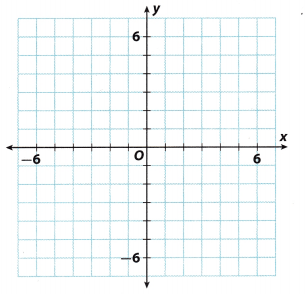Step 1: Translate point K
Count 3 units to the right and 4 units up and plot point K'(0, 6).

Step 2: Translate point L.
Count 3 units to the right and 4 units up and plot point L'(5, 6).

Step 3: Translate point M.
Count 3 units to the right and 4 units up and plot point M'(3, 1).

Step 4: Translate point N
Count 3 units to the right and 4 units up and plot point N'(-1, 4)

b. On the same coordinate grid, graph the image of quadrilateral KLMN after a translation of 3 units to the right and 4 units up.
Connect K’, L’, M’ and N’ to form quadrilateral K’L’M’N’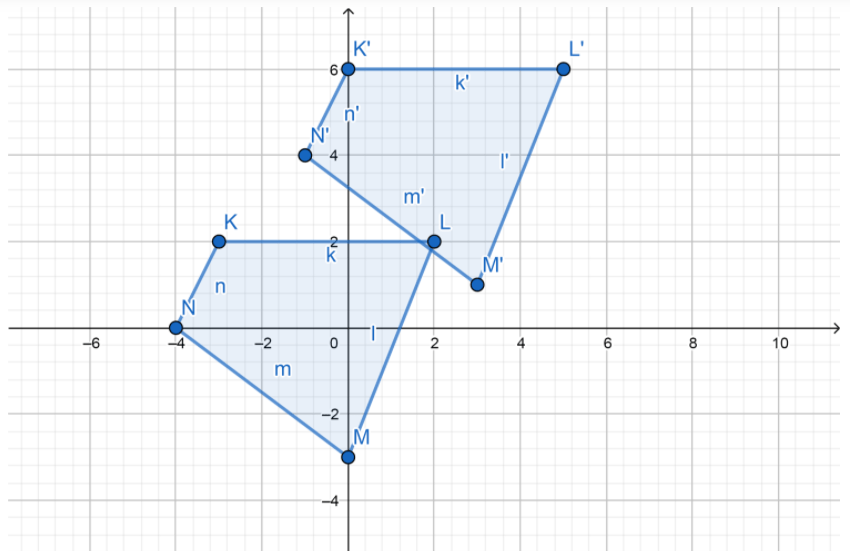c. Which side of the image is congruent to side $$\overline{L M}$$?
Name three other pairs of congruent sides.
$$\overline{L^{\prime} M^{\prime}}$$ is conguent to $$\overline{L M}$$.
$$\overline{K^{\prime} L^{\prime}}$$ is conguent to $$\overline{K L}$$.
$$\overline{M^{\prime} N^{\prime}}$$ is conguent to $$\overline{M N}$$.
$$\overline{K^{\prime} N^{\prime}}$$ is conguent to $$\overline{K N}$$.

Draw the image of the figure after each translation.

Question 9.
4 units left and 2 units down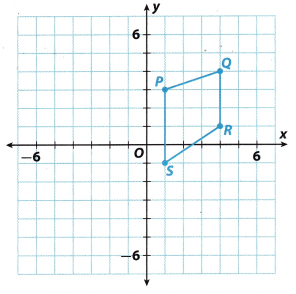Step 1: Translate point P.
Count 4 units to the left and 2 units down and plot point P'(-3, 1).

Step 2: Translate point Q.
Count 4 units to the left and 2 units down and plot point Q'(0, 2).

Step 3: TransLate point R.
Count 4 units to the left and 2 units down and plot point R'(0, 1).

Step 4: Translate point S.
Count 4 units to the left and 2 units down and plot point S'(-3, -3).

Step 5: Connect P’, Q’, R’ and S’ to form quadrilateral P’Q’R’S’.Connect P'(-3, 1), Q'(0, 2), R'(0, -1) and S'(-3, -3) to form quadriLateraL P’Q’R’S’.

Question 10.
5 units right and 3 units upStep 1: Translate point A.
Count 5 units to the right and 3 units up and plot point A'(0, 4).

Step 2: Translate point B.
Count 5 units to the right and 3 units up and plot point B'(3, 5).

Step 3: Translate point C.
Count 5 units to the right and 3 units up and plot point C'(3, 1).

Step 4: Translate point D.
Count 5 units to the right and 3 units up and plot point D'(0, 0).

Step 5: Connect A’, B’, C’ and D’ to form quadrilateral A’B’C’D’.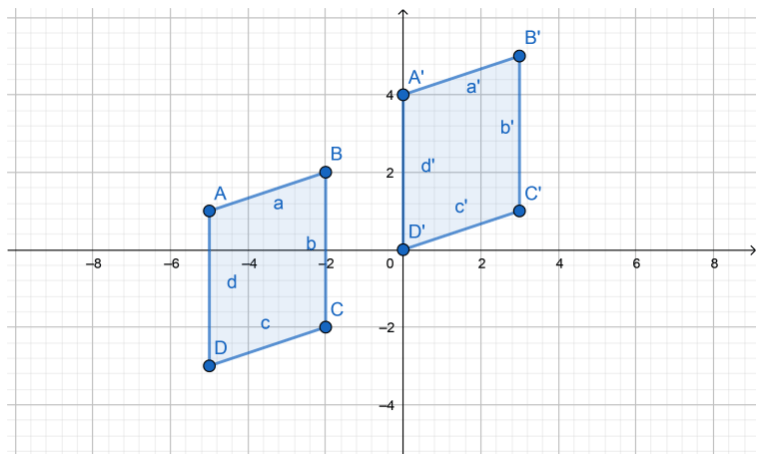Question 11.
The figure shows the ascent of a hot air balloon. How would you describe the translation?From the picture we can see that the balloon moved 4 units to the right and 5 units up.

Question 12.
Critical Thinking Is it possible that the orientation of a figure could change after it is translated? Explain.
No, it’s not possible. A translation is a transformation when a figure moves to another location without any change in size or orientation.

H.O.T. Focus on Higher Order Thinking

Question 13.
a. Multistep Graph triangle XYZ with vertices X(-2, -5), Y(2, -2), and Z(4, -4) on the coordinate grid.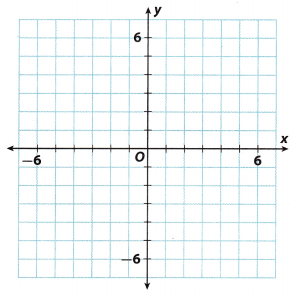Step 1: Translate point X.
Count left 3 units and up 6 units and plot point X'(-5, 1).

Step 2: Translate point Y
Count left 3 units and up 6 units and plot point Y'(-1, 4).

Step 3: Translate point Z.
Count left 3 units and up 6 units and plot point Z'(1, 2).

b. On the same coordinate grid, graph and label triangle X’Y’Z’, the image of triangle XYZ after a translation of 3 units to the left and 6 units up.
Connect X’, Y’ and Z’ to form triangle X’Y’Z’c. Now graph and label triangle X”Y”Z”, the image of triangle X’Y’Z’ after a translation of 1 unit to the left and 2 units down.
Step 1: Translate point X’.
Count left 1 unit and down 2 units and plot point X”(-6, -1)

Step 2: Translate point Y’
Count left 1 unit and down 2 units and plot point Y”(-2, 2)

Step 3: Translate point Z’.
Count left 1 unit and down 2 units and plot point Z”(0, 0).

Step 4: Connect X”, Y” and Z” to form triangle X”Y”Z”.

d. Analyze Relationships How would you describe the translation that maps triangle XYZ onto triangle X”Y”Z”?
After a translation of 4 units up and 4 units to the left, we get triangle X”Y”Z”, the image of the triangle XYZ.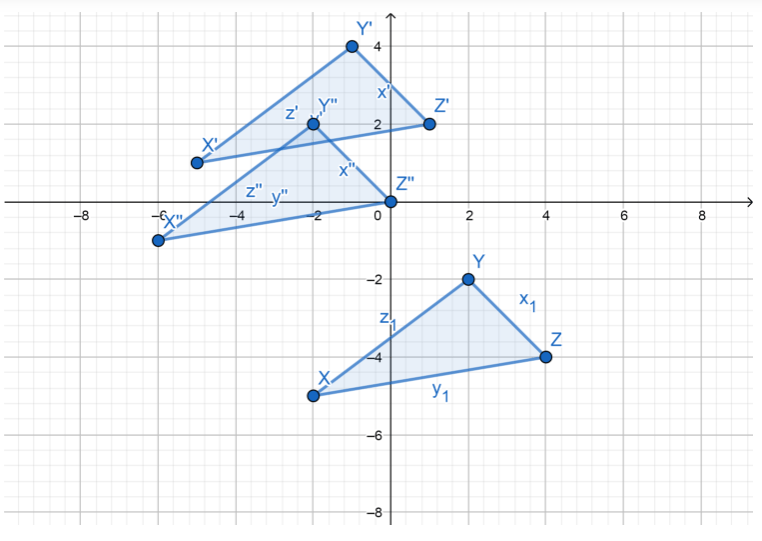Question 14.
Critical Thinking The figure shows rectangle P’Q’R’S’, the image of rectangle PQRS after a translation of 5 units to the right and 7 units up. Graph and label the preimage PQRS.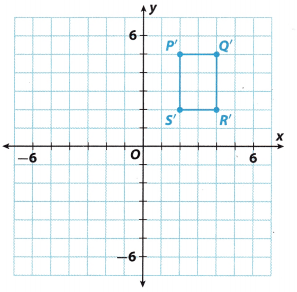The figure shows us the image of rectangle PQRS after a translation of 5 units to the right and 7 units up. We need to find the preimage of the rectangle. So, we will come back 5 units to the left, and 7 units down.
Count 5 units to the left and 7 units down and plot point P(-3, -2).
Count 5 units to the left and 7 units down and plot point O(-1, -2).
Count 5 units to the left and 7 units down and plot point R(-1, -5).
Count 5 units to the left and 7 units down and plot point S(-3, -5).
Connect P, Q, R and S and form a rectangle PQRS.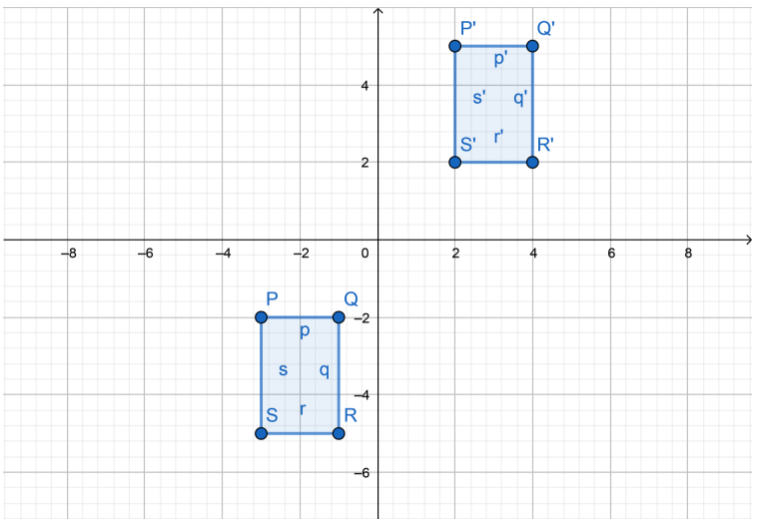P(-3, -2)
O(-1, -2)
R(-1, -5)
S(-3, -5)

Question 15.
Communicate Mathematical Ideas Explain why the image of a figure after a translation is congruent to its preimage.